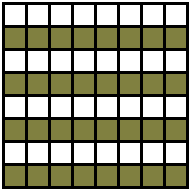# Tiling a Square with T-, L-, and a Square Tetrominoes

Both L- and T-tetrominoes tile a chessboard. It takes 16 tiles of each kind. The question I wish to ask is whether

1. Is it possible to cover a chessboard with 15 T-tetrominoes and a square one?
2. Is it possible to cover a chessboard with 15 L-tetrominoes and a square one?

It is known that a straight tromino (or even three of them) could not be replaced with square tiles. What is happening with T- and L-tetromino tilings?

Solution### Solutions

1. Is it possible to cover a chessboard with 15 T-tetrominoes and a square one?In the common coloring of a chessboard, a T-tetromino covers an odd number of white (1 or 3) and an odd number (also, 1 or 3) of dark squares. It follows that an odd number (15 in particular) will cover an odd number of white and an odd number of dark squares. A square tile would add 2 white and 2 dark squares to such a tiling, leaving the totals odd. But the number of white and dark squares on the board is even, implying that no such tiling is possible.

1. Is it possible to cover a chessboard with 15 L-tetrominoes and a square one?

The answer is again, No. Now we color the board differently:The argument is exactly the same. In the new coloring, an L-tetromino covers an odd number of cells of the same color, whereas a square tetromino always covers 2 cells of each color.

### References

1. Anany and Maria Levitin, Algorithmic Puzzles (Oxford University Press, 2011), #33(f)### Related materialRead more...

• Covering A Chessboard With Domino
• Dominoes on a Chessboard
• Tiling a Chessboard with Dominoes
• Vertical and Horizontal Dominoes on a Chessboard
• Straight Tromino on a Chessboard
• Golomb's inductive proof of a tromino theorem
• Tromino Puzzle: Interactive Illustration of Golomb's Theorem
• Tromino as a Rep-tile
• Tiling Rectangles with L-Trominoes
• Squares and Straight Tetrominoes
• Covering a Chessboard with a Hole with L-Trominoes
• Tromino Puzzle: Deficient Squares
• Tiling a Square with Tetrominoes Fault-Free
• Tiling a Rectangle with L-tetrominoes
• Tiling a 12x12 Square with Straight Trominoes
• Bicubal Domino
•